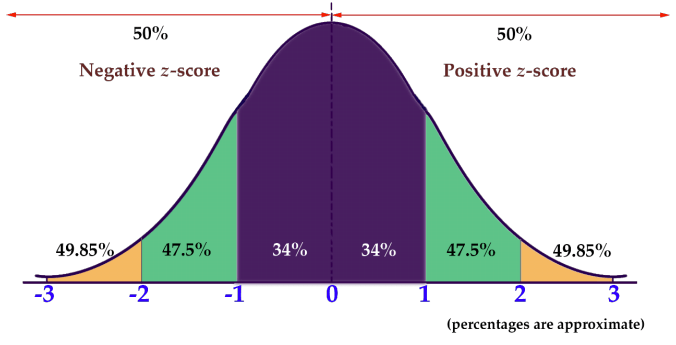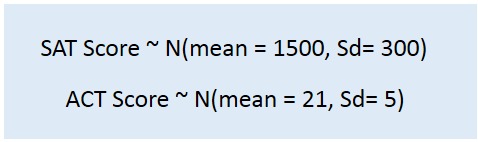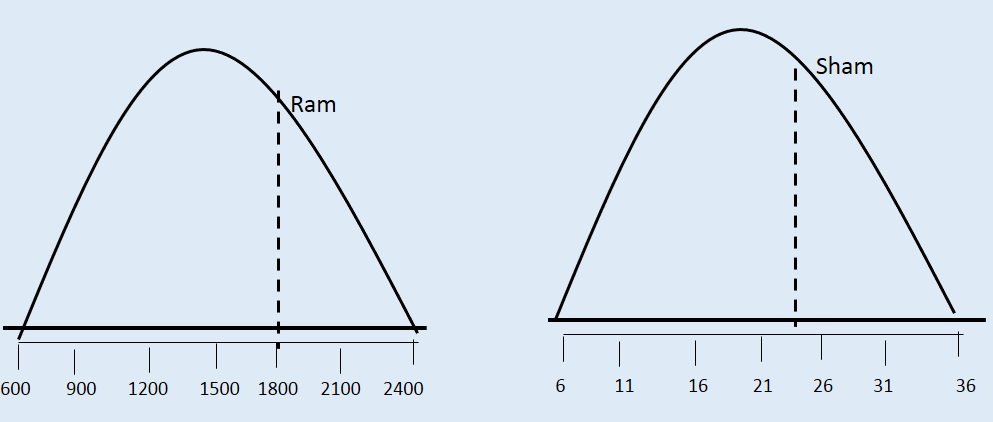### Z-score or Standardized Score

#### What is Z-score and how to calculate them?

• A Standardized Score (Z-Score) is useful to know how many standard deviations an element falls from the mean. A z-score can be calculated from the following formula.where z is the z-score, X is the value of the element, μ is the population mean, and σ is the standard deviation.

• Z-score will help to understand a specific observation is common or exceptional in your study.
• As mean is the middle point. So, negative z-score represent values below the mean. While positive z-score represent values above the mean
• If you add all Z-Score you will get a value 0 because positive and negative z-score will cancel out each other.
• If your data is extremely right skewed then probably you will get large positive Z-Score. On the other way, if distribution is left skewed then you will get large negative Z-Score
• Z-Score of Mean is 0 as it is the middle value
• If value of |Z| is greater than 2 then we can tell a distribution is unusual or exceptional.

#### Why Z-Score is needed?

• Sometimes, in your statistical analysis you want to figure out a specific observation is common or exceptional case. Then Z-score will help to understand the standard deviation it falls below or above the mean.

#### Bell Shaped Distribution and Empirical Rule

If distribution is bell shape then it is assumed that about 68% of the elements have a z-score between -1 and 1; about 95% have a z-score between -2 and 2; and about 99% have a z-score between -3 and 3.#### Let’s take an example why Z-Score are useful?

A person is having two sons. He wants to know who scored better on their standardized test with respect to the other test takers. Ram who earned an 1800 on his SAT or Sham who scored a 24 on his ACT Exam ?Here we cannot simply compare and tell who has done better as they are measured in different scale.So, his father will be interested to observe how many standard deviation of their respective mean of their distribution Ram and Sham score.

Ram = (1800- 1500) / 300 =1 standard deviation above the mean

Sham = (24 – 21 ) / 5= 0.6 standard deviation above the mean

Now his father can conclude Ram indeed did a better score than Sham.

If it is still not clear to you and want to explore more then can go to the below sites and have a look.

https://en.wikipedia.org/wiki/Standard_score

https://statistics.laerd.com/statistical-guides/standard-score-2.php

http://stattrek.com/statistics/dictionary.aspx?definition=z%20score

http://www.nku.edu/~statistics/212_Using_the_Empirical_Rule.htm

You can find some other related questions about Z-Score in Quora

https://www.quora.com/Does-the-empirical-Rule-include-4-standard-deviations

https://www.quora.com/While-calculating-a-z-score-what-do-you-do-when-standard-deviation-is-zero